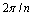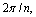1800-1023-196

+91-120-4616500

# Crystallography

## Crystallography

“The branch of science that deals with the study of structure, geometry and properties of crystals is called crystallography”.

(1) Symmetry in Crystal: A crystal possess following three types of symmetry,

(i) Plane of symmetry: It is an imaginary plane which passes through the centre of a crystal can divides it into two equal portions which are exactly the mirror images of each other.(ii) Axis of symmetry : An axis of symmetry or axis of rotation is an imaginary line, passing through the crystal such that when the crystal is rotated about this line, it presents the same appearance more than once in one complete revolution i.e., in a rotation through 360°. Suppose, the same appearance of crystal is repeated, on rotating it through an angle of 360°/n, around an imaginary axis, is called an n-fold axis where, n is known as the order of axis. By order is meant the value of n inso that rotation throughgives an equivalent configuration.(iii) Centre of symmetry: It is an imaginary point in the crystal that any line drawn through it intersects the surface of the crystal at equal distance on either side.Only simple cubic system have one centre of symmetry. Other system do not have centre of symmetry.

The total number of planes, axes and centre of symmetries possessed by a crystal is termed as elements of symmetry.

A cubic crystal possesses total 23 elements of symmetry.

Plane of symmetry (3 + 6) = 9

Axes of symmetry (3 + 4 + 6) = 13

Centre of symmetry (1) = 1

Total symmetry = 23

## NEET & AIIMS Exam Sample Papers

 AIIMS SAMPLE PAPERS View More NEET SAMPLE PAPERS View More